Category
Categories
Sort By
• Latest
• Year

# Knowledge Distillation in Deep Learning

28 / Jan / 2022 by Prashant Brahmbhatt 0 comments

There were times when we had our teachers help us out in solving difficult problems. We took our problem to someone who could understand it better and can give a good overview which enabled us then to solve the problem.

The same can be done in deep learning as well. Yes, we can actually teach a model from another model. Superficially it may sound like Transfer Learning but it isn’t. These are fairly different concepts. In this post, we are going to discuss the entire process.

The explanation is aimed at giving minimal but sufficient understanding of distillation and implement when needed. The actual paper for this concept can be read here.

But why would we want to learn from another model? If we are to train for learning process can’t we just train on the data itself?

There are a couple of situations in which we might want to use distillation. We’ll discuss the advantages later in the post.

### The Teacher and Student

In the distillation process, the model which is to learn is termed as the Student model while the model from which we learn is referred to as the Teacher model.

The teacher is usually a more complex model that has more ability and capacity for knowledge from any given data. A large amount of data can be quite easily understood by a model containing hundreds of layers. It is also referred to as the ‘Cumbersome’ model. To gather, the same amount of knowledge for a simple model is sometimes impossible due to variations or the large volumes of data.

### The Teaching

We are talking about teaching the model but how is that ‘teaching’ supposed to happen technically?

Consider solving a problem from our math book, we take one problem, and try to solve it ourselves. Then we check the back of the book for answers but we find that we got the answer incorrect! What now? The answer helped us to know that what we did was incorrect but we’re not really sure what went wrong, we don’t know how close we were, or did we get confused among some steps? Now our teacher can help us with that, a simple nudge in the right direction can get us on track. Or if the teacher solves a couple of such problems we can compare our solution with that of our teacher and can get a better idea of the problem.

The same principle is applied in deep learning, the student model is exposed to the answers of the teacher model. We will give the outputs calculated by the cumbersome model to the simpler model.

But isn’t that kinda same as giving it the actual targets, the real ones?

No! And there’s a catch as well. We are not going to provide the final predictions of the teacher model but the logits. Logits here are the outputs obtained before the final activation layer. So the sum of the logit prediction won’t necessarily sum up to 1. If earlier that model was seeing only the targets as `[1,0]` , here it will see something like `[12.7777, -3.99999]` along with the true targets. These scores of the cumbersome model provided in addition are known as soft targets.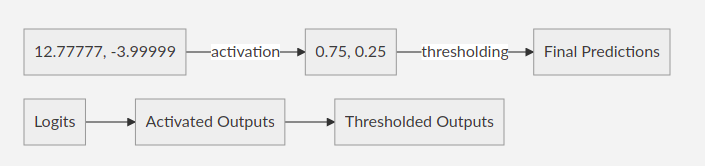When the classes are quite similar or way too different the soft targets will give the model a better idea about such scenarios. Even giving the softmax activated outputs would be slightly better than the targets but logits are what we will work with as proposed in the actual paper.

For the training, we could use the same set that the teacher model used for the training, or we could have a separate transfer set if we wish.

During the implementations what we do is just strip out the final activation layer of the teacher model, then get its outputs and just feed them to the student along with the actual targets.

### The Temperature

Temperature is a parameter that is used to tweak the distribution of the soft targets. The general formula for the softmax function is a simpler version of the same formula with temperature: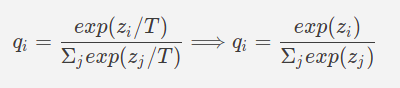The T in the formula stands for that temperature, generally, it is taken as 1 while calculating the softmax outputs.

The temperature value affects the sharpness of the distribution of values. The higher the value of the temperature the softer the distribution becomes. We can observe this behavior in the below plot for some sample values.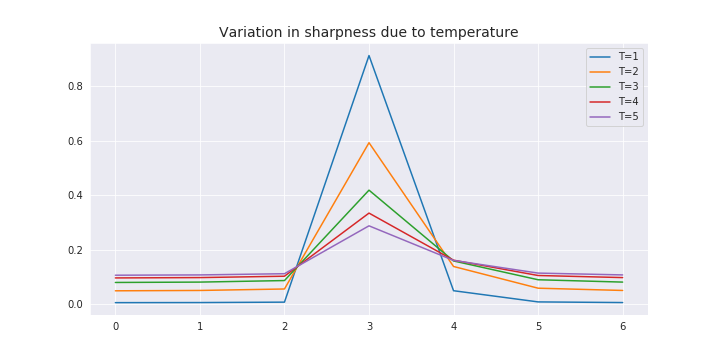As we can see how increasing the temperature has brought down the difference between the sample values. We can use different values of the temperature for our purposes, just another hyperparameter that we would need to tune. Although, the different temperature value is only to be used during the training of the student model, once the model is done training, the predictions from that model are to be made using the temperature value as 1.

### The Loss Function

We would use the weighted average of two different objective functions.

• The first loss function would be the cross-entropy with the soft targets
• The second loss function would be cross-entropy with the correct labels

The loss calculation for the first function has to be computed using the same temperature as was used to compute the logits from the cumbersome model.

### Lambda

The parameter λ is used as the weight parameter for the final loss function. The weighted loss function would be something like the below equation: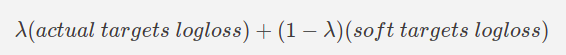Preferably, higher importance or weight is given to the soft targets which of course, can be controlled to cater to our specific problem. We could use something like 0.1 for the value of λ which would mean 90% contribution of soft-target loss while 10% loss from the true target loss. Again, it’s something we would need to play with.

Process for implementation would look something like this,We would first create the logits from the teacher model and save them into a numpy file.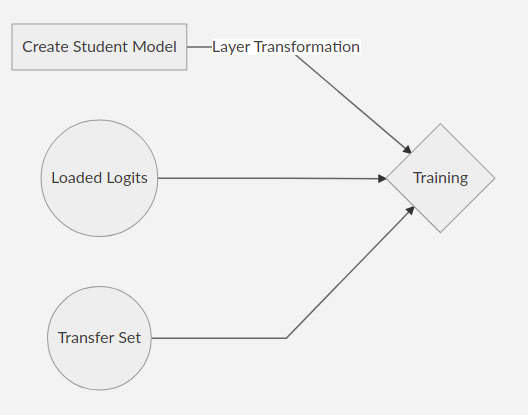Then a student model would be created and modifications in the last few layers would be needed for the training on the transfer set and loading the saved logits.

After the transformation, there would be two activation layers rather than just one. In one of the layers, the normal activations would be done while in the other the soft activations would be performed.

The transformation in the `Keras API` would look something like this:

Before Transformation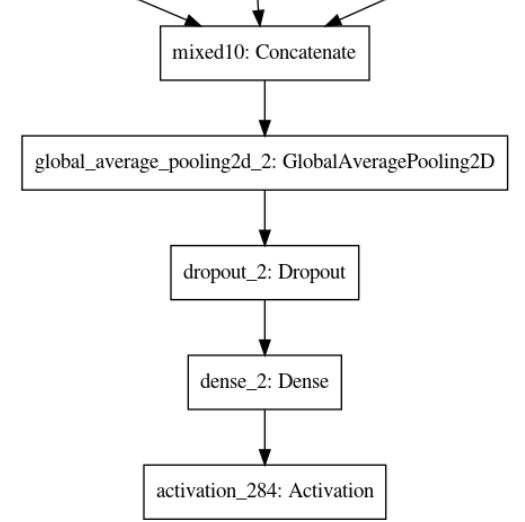After Transformation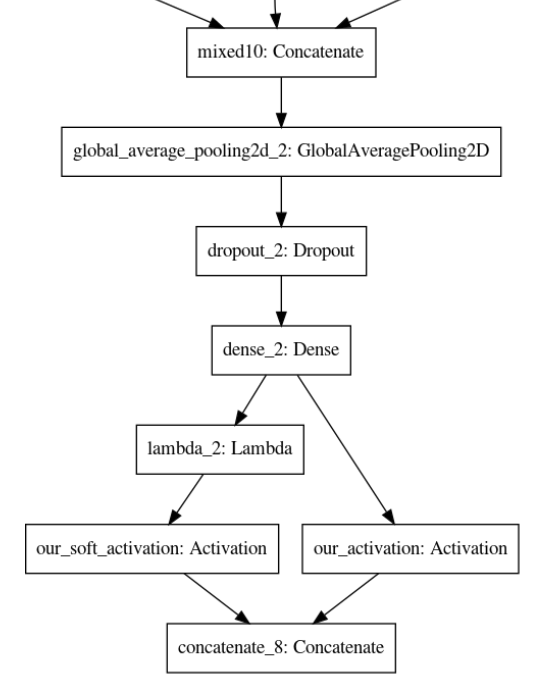Later for using the trained model, if we have to load the weights, first we will have to define the model in the exact same way with all the layer transformations and temperature for it to work.

Alternatively, we can just load the entire model rather than just weights for which we would not have to define anything, making our life easier.

### Ensemble using Distillation

Knowledge Distillation is very suitable for solving problems through the ensemble. In some scenarios where the number of classes is large, even a single cumbersome model is not quite able to encompass all the information while also covering the subtle detailed differences.

Using ensemble as one of the workarounds, we can create multiple specialized models which only focus on some of the classes which are similar to each other and can be confused with during the predictions.

Once the specialized models are created we can use distillation to encode all of the information from different models into just one model. Rather than using all the models to get ensemble outputs, we could then just use that one distilled model containing the knowledge of all the other models.

### Pros and Cons

Every methodology has its advantages as well as certain limitations and so does knowledge distillation, so let’s discuss them starting with the Pros.

#### Pros

There are quite a few advantages of using knowledge distillation, some of them are:

• Highly effective for ensemble techniques.

• Using distillation can save you a lot of space as well, as in the case of an ensemble where we could have just one model rather than keeping all the ensemble models to get the outputs.

• Can identify classes, not in the data. A simpler model can also give satisfactory results on the classes that it may not have seen at all in the transfer set used in training, provided that the cumbersome model has seen those classes.

• Helpful in getting structure from complex data for simpler models which can have trouble doing that on their own. Sometimes even when a simpler model has enough capacity for large knowledge, it may still struggle with extracting the useful features. The cumbersome model can help it find those complex features.

• A mystery model about which we don’t have a lot of information can be cloned to an architecture of our choice. This was the use case that we had to face in one of our projects. We had a better model but no idea about its data and other parameters. Our simpler models were struggling. So we used that model to help our simpler model in performing better and it actually worked.

#### Cons

There aren’t really any great downsides of distillations that we noticed but just a couple of caution points.

• It is a little complex to implement correctly. It is not actually a con but something that we just faced. We tried implementing it using Keras. The example problem given in the Keras documentation works but we were not able to make it work using a generator to get data from local directories.

• Careful understanding of the problem. Before we implement distillation we need to understand our problem statement and how we would fit the distillation and would or would it not help our case.

• May require some more tuning to get a balanced performance. Distillation has two more parameters, Lambda and Temperature that we need to tune to get the process working correctly for our use case.

### Conclusion & Use case

The concept of knowledge distillation was proved to be useful in one of our client projects at To The New. The client had an existing image classification model whose performance was hard to beat but the model was heavy and was not best suited for real-time inferencing. So we needed to create another image classification that could replicate similar performance to that of the existing model but also has a relatively smaller architecture so its deployment overhead is significantly less compared to the prior.

We were not able to replicate the performance of the existing model despite preparing several datasets and combinations of hyperparameters but using knowledge distillation we were able to achieve that performance of the existing model.

Knowledge Distillation is very useful in other similar situations and an alternate ready to use official implementation is also available using the Keras API available here.

FOUND THIS USEFUL? SHARE IT

### Tag -

Knowledge Distillation
Services